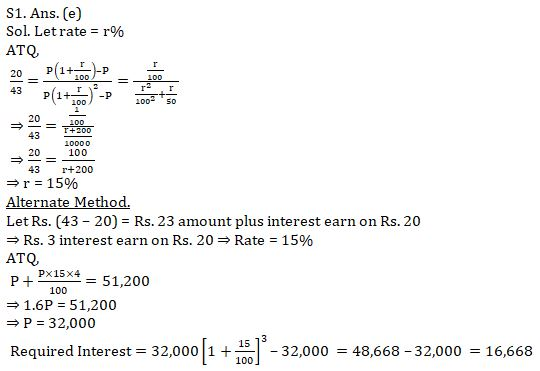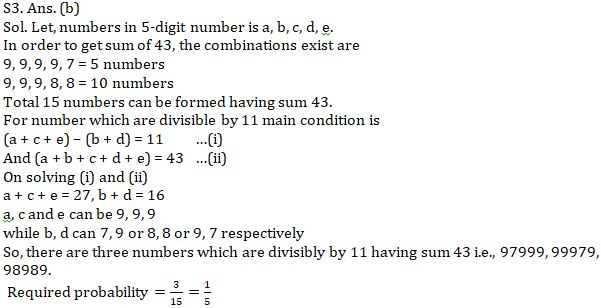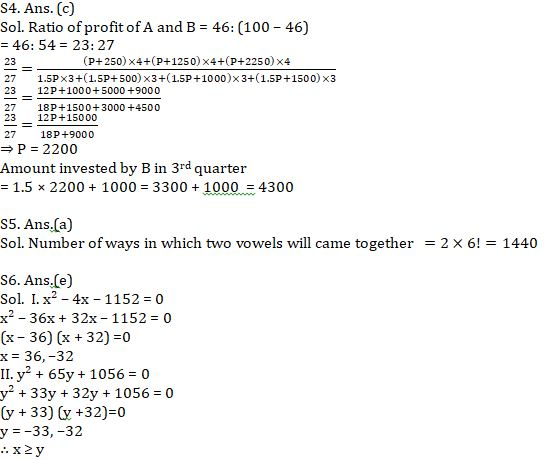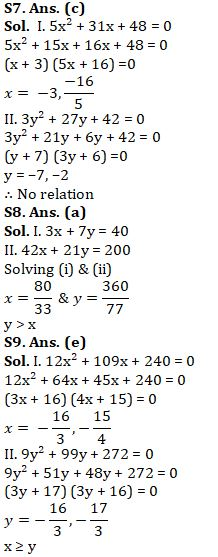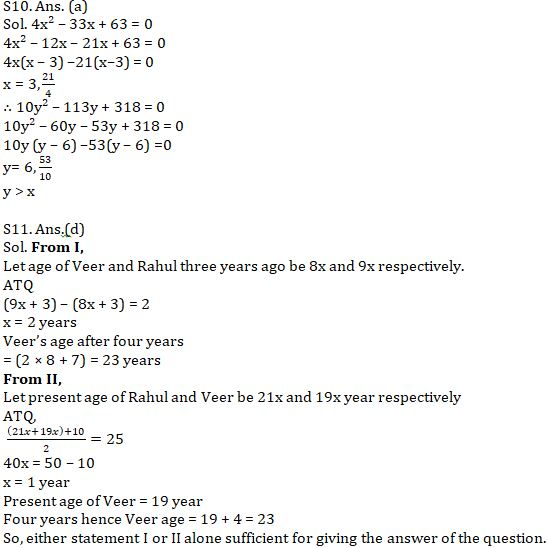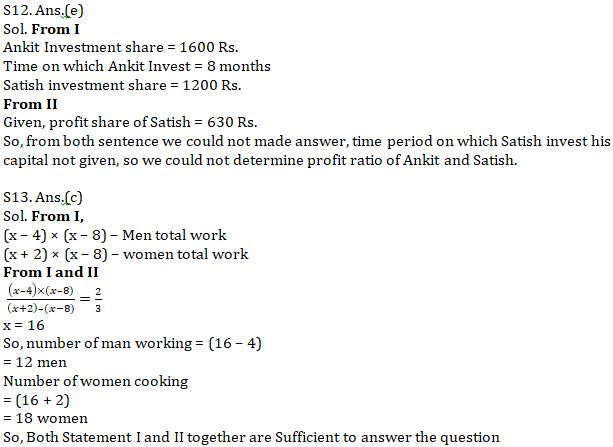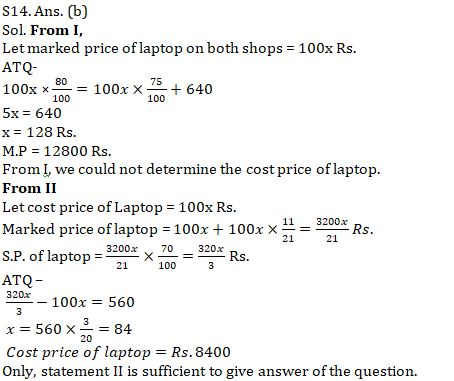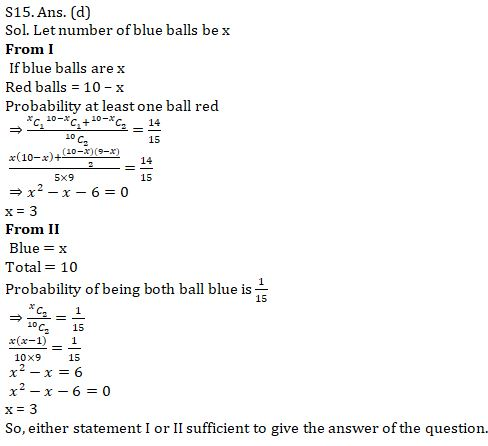Latest Banking jobs   »   Quantitative Aptitude Quiz For SBI Clerk...

# Quantitative Aptitude Quiz For SBI Clerk Mains 2021- 31th July

Q1. Raman invested Rs. P in scheme ‘X’ which offers some rate at compound interest p.a. If ratio of interest earned by him after first year to second year from scheme ‘X’ is 20 : 43 while if Raman invested Rs. P in scheme ‘Q’ which offers same rate but at simple interest then after 4 years sum amounts to Rs. 51,200, then find the interest he can earn if he invested Rs. P for 3 years in scheme ‘X’.
(a) 16,248
(b) 16,868
(c) 15,548
(d) 14,656
(e) 16,668

Q2. A circle is inscribed in a square. If the difference between area of the square and circle is 262.5 cm², then find the area of the rectangle whose perimeter is same as that of circle while length of rectangle is 20% more than the breadth of rectangle (in cm²)
(a) 1500
(b) 1400
(c) 700
(d) 750
(e) 3000

Q3. Sum of digits of a 5-digit number is 43. Find the probability that such a number is divisible by 11?
(a) 1/3
(b) 1/5
(c) 2/5
(d) 2/15
(e) 2/3

Q4. ‘A’ and ‘B’ started a business together. ‘A’ invested (P + 250) initially while ‘B’ invested ‘1.5P’ initially. ‘A’ invested Rs. 1000 more after every 4 months while ‘B’ invested Rs. 500 more after every quarter. After an year ‘A’ got 46% of total profit then find the amount invested by ‘B’ in 3rd quarter?
(a) 3300
(b) 3800
(c) 4300
(d) 4800
(e) 5300

Q5. In how many ways word ‘DELIGHT’ can be written such that both vowels will always come together.
(a) 1440
(b) 720
(c) 1569
(d) 7560
(e) 3360

(a) x < y
(b) x ≤ y
(c) x = y or no relation can be established between x and y.
(d) x > y
(e) x ≥ y
Q6. I. x² – 4x – 1152 = 0
II. y² + 65y + 1056 = 0

Q7. I. 5x² + 31x +48 = 0
II. 3y² + 27y + 42 = 0

Q8. I. 3x + 7y = 40
II. 42x + 21y = 200

Q9. I. 12x² + 109x + 240 = 0
II. 9y² + 99y + 272 = 0

Q10. I. 4x² – 33x + 63 = 0
II. 10y² – 113y + 318 = 0

Directions (11-15): The following questions are accompanied by two statements (I) and (II). You have to determine which statements(s) is/are sufficient/necessary to answer the questions.
(a) Statement (I) alone is sufficient to answer the question but statement (II) alone is not sufficient to answer the questions.
(b) Statement (II) alone is sufficient to answer the question but statement (I) alone is not sufficient to answer the question.
(c) Both the statements taken together are necessary to answer the questions, but neither of the statements alone is sufficient to answer the question.
(d) Either statement (I) or statement (II) is sufficient to answer the question.
(e) Statements (I) and (II) together are not sufficient to answer the question.

Q11. Find Veer’s age four years hence from present?
(I) Ratio between Veer’s age and Rahul’s age, three years ago was 8 : 9, while Veer is two years younger than Rahul.
(II) Ratio between present age of Rahul and Veer is 21: 19, while five year hence average age of Rahul and Veer will be 25 years.

Q12. What will be profit share of Ankit out of total profit?
(I) Ankit Invest Rs. 1600 for 8 months and Satish joined him after third month with capital of Rs. 1200.
(II) Satish got Rs. 630 as profit share.

Q13. How many man and women working on this task?
(I) (x – 4) man work for first (x – 8) days, after that (x+2) woman complete the remaining work in (x – 8) days.
(II) Ratio between work did by men to work did by women is 2 : 3.

Q14. Find the cost price of laptop if cost price of laptop on both shops is same?
(I) Marked price of laptop on two shops A and B is same. Shops ‘A’ allows 20% discount and shop B allows 25% discount on laptop. A man purchased laptop from shop B, if man spend Rs. 640 more, he could purchase the same laptop from shop A.
(II) Marked price of laptop is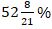more than its cost price. If discount of 30% allows on M.P. of laptop, shopkeeper made a profit of Rs. 560.

Q15. There are some red and some blue balls in a bag. Find how many blue balls in the bag.
(I) Total number of balls in bag is ten. If selecting two balls at random probability of being at least one ball red is 14/15.
(II) Total number of balls in bag is ten. Selecting two balls from out of total balls at random, probability of being both ball blue is 1/15 .

Solutions